# Probability that a given observation is part of a bootstrap sample?

“The bootstrap is a widely applicable and extremely powerful statistical tool that can be used to quantify the uncertainty associated with a given estimator or statistical learning method.” This is how Section 5.2 of the book An Introduction to Statistical Learning, written by Gareth James, Daniela Witten, Trevor Hastie and Robert Tibshirani, begins. Bootstrap can be used to estimate the standard errors of the coefficients from a linear regression and is the conceptual basis for some tree ensemble algorithms.

This post is not devoted the bootstrap itself but rather about understanding the often encountered statement:

On average, each bootstrap sample makes use of around two-thirds of the observations.

What does this mean and why it is true?

Recall that a bootstrap sample of $$n$$ observations is just a to randomly choose $$n$$ observations with repetition.

Aim: Derive the probability that a given observation is part of a bootstrap sample.

To do this we are going to follow the lines of the Exercise 5.4.2 of the book An Introduction to Statistical Learning. We are going to use Python despite the fact that this reference uses mainly R.

## Solution of Excercise 5.4.2

Suppose that we obtain a bootstrap sample from a set of $$n$$ observations $$x_1, …, x_n$$.

(a) What is the probability that the first bootstrap observation is not the $$j$$-th observation from the original sample?

Let us denote this probability by $$_j$$. As the probability of selecting a particular $$x_j$$ from the set $$x_1, …, x_n$$ is $$1/n$$, then the desired probability is

$\begin{equation*} \pi_j = 1-\frac{1}{n} \end{equation*}$

(b) What is the probability that the second bootstrap observation is not the $$j$$-th observation from the original sample?

Well, it is not hard to see that, as the selections are independent then, this probability is again $$_j$$.

(c) Argue that the probability $$p_j(n)$$ that the $$j$$-th observation is not in the bootstrap sample is $$(1 − 1/n)^n$$.

It is straight forward to see from (a) and (b) that this probability is given by

$\begin{equation*} p_j(n)= \prod_{i=1}^n \pi_j = \left(1-\frac{1}{n}\right)\left(1-\frac{1}{n}\right)\cdots\left(1-\frac{1}{n}\right) =\left(1-\frac{1}{n}\right)^n. \end{equation*}$

Let us define a function which computes this probability.

def probability(n):
return (1 - 1/n)**n

(d) When $$n = 5$$, what is the probability that the $$j$$-th observation is in the bootstrap sample?

# This is simply:
1 - probability(5)
0.6723199999999999

(e) When $$n = 100$$, what is the probability that the $$j$$-th observation is in the bootstrap sample?

1 - probability(100)
0.6339676587267709

(f) When $$n = 10000$$, what is the probability that the $$j$$-th observation is in the bootstrap sample?

1 - probability(1e4)
0.6321389535670295

(d) Create a plot that displays, for each integer value of $$n$$ from $$1$$ to $$100,000$$, the probability that the $$j$$-th observation is in the bootstrap sample. Comment on what you observe.

We first do it for $$n$$ from $$1$$ to $$50$$:

import numpy as np
import matplotlib.pyplot as plt
plt.style.use('seaborn')

%matplotlib inline

def generate_plot(sample_size_max):

sample_sizes = np.arange(sample_size_max, dtype=float)

probability_sequence = [1 - probability(n+1) for n in sample_sizes]

plt.figure(figsize=(9, 7))
plt.plot(sample_sizes, probability_sequence, marker='o')
plt.plot(sample_sizes, probability_sequence, color='red')
plt.title('Probability Sequence')
plt.xlabel('Sample Size')
plt.ylabel('probability the j-th observation is in the bootstrap');
generate_plot(50);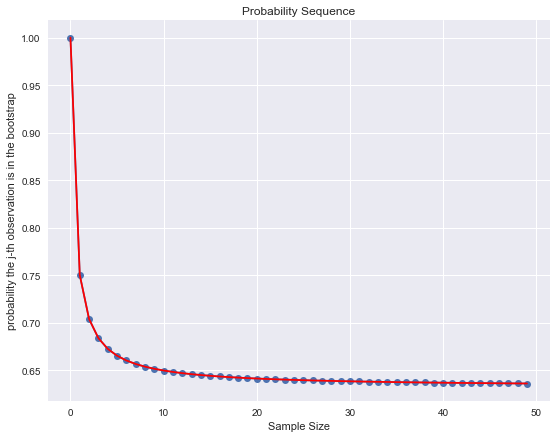Now we do it for $$n$$ from 1 to 100,000:

generate_plot(1e5);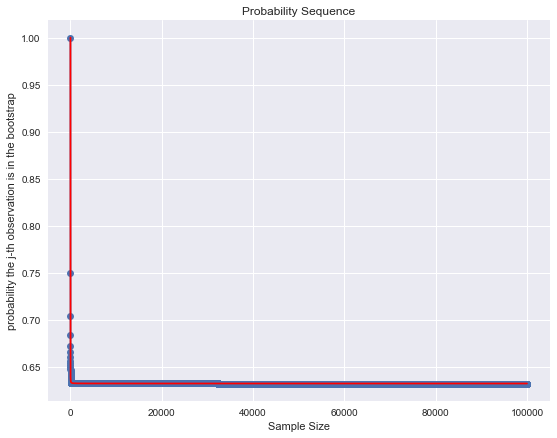Clearly this probabilities are converging! But, to which value?

Recall that the exponential function satisfies:

$\begin{equation*} e^x = \lim_{n\rightarrow \infty} \left(1+\frac{x}{n}\right)^n \end{equation*}$

Hence, we easily see that

$\begin{equation*} p_j := \lim_{n\rightarrow \infty}p_j(n) = e^{-1} \approx 0.3678794 \end{equation*}$

Therefore the limit as $$n$$ increases of the probability the $$j$$-th observation is in the bootstrap is:

1 - np.exp(-1) # Which is roughly 2/3.
0.6321205588285577

Let us plot this:

generate_plot(50)
plt.axhline(y=1 - np.exp(-1) , color='g', linestyle='--');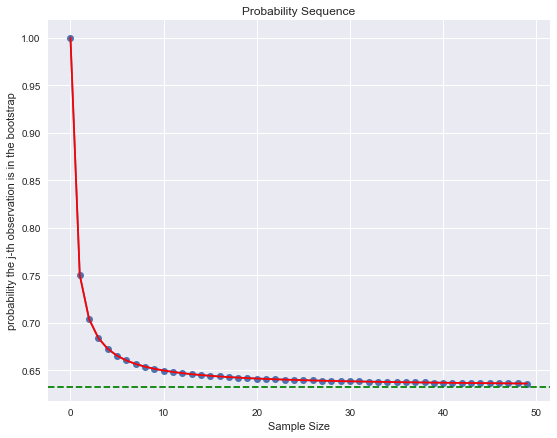generate_plot(1e5)
plt.axhline(y=1 - np.exp(-1) , color='g', linestyle='--');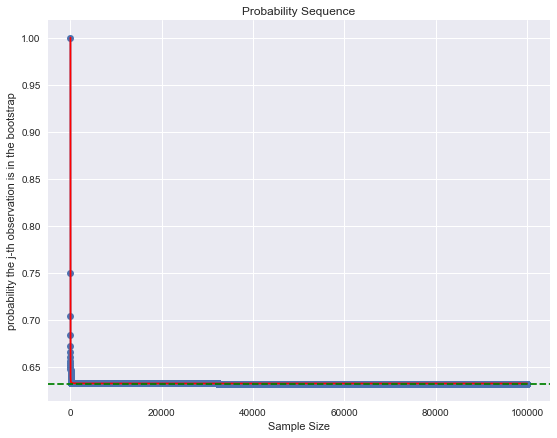(h) We will now investigate numerically the probability that a bootstrap sample of size $$n = 100$$ contains the $$j$$-th observation. Here $$j = 4$$. We repeatedly create bootstrap samples, and each time we record whether or not the fourth observation is contained in the bootstrap sample.

We write this function as:

def get_probability(num_iterations, n, j):

sample = [
(np.random.choice(n, n) == j).sum() > 0
for i in range(0, num_iterations)
]

sample = np.array(sample)

return sample.mean()

num_iterations = int(1e5)
n = 100
j = 4

sample_mean = get_probability(num_iterations, n, j)
sample_mean
0.63511

This value is quite close to $$1-e^{-1}$$.

abs(sample_mean - (1 - np.exp(-1)))
0.0029894411714422864

Let us go a step further as do this computation many times!

def simulation(n, j, N):

simulation_array = [
get_probability(num_iterations, n, j)
for i in range(0, N)
]

return np.array(simulation_array)

We run the simulations:

simulation_array = simulation(n, j, N=500)

Let us see the histogram of the samples:

plt.figure(figsize=(9, 7))
plt.hist(simulation_array, bins=30)
plt.axvline(simulation_array.mean(), color='red', linestyle='--', label='simulation mean')
plt.axvline(1 - np.exp(-1), color='purple', linestyle='--', label='1 - exp(-1)')
plt.legend()
plt.title(f'Histogram:  Bootstrap sample of size n=100 contains the {j}-th observation');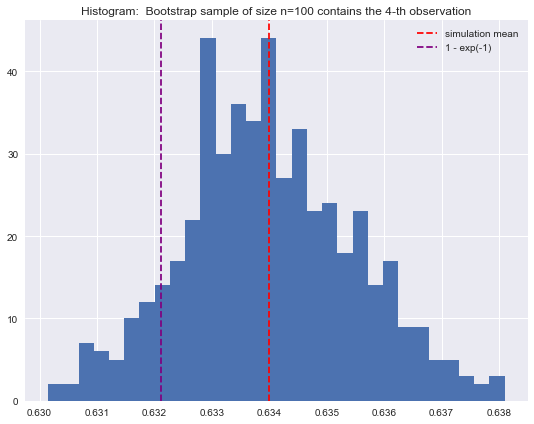If we compute the mean:

simulation_array.mean()
0.63398918

and compare it with

1 - probability(n)
0.6339676587267709
abs(simulation_array.mean() - (1 - probability(n)))
2.1521273229185844e-05

We get the expected result!